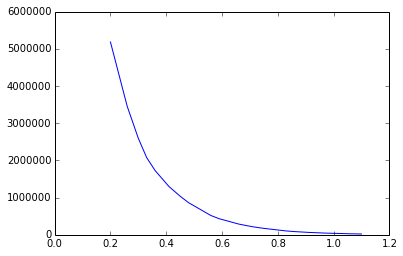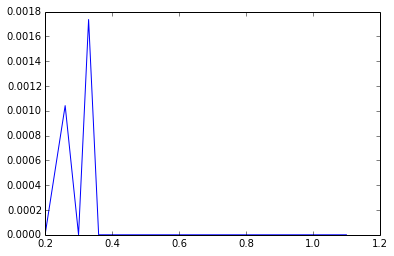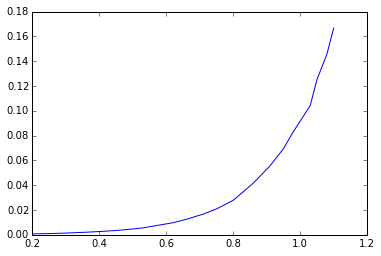# Chapter 5 : GROUND WATER WELL IRRIGATION¶

## Example 5.1 pg : 277¶

In :
import math
#design an open wellin fine sand

#Given
Q = 0.003;        				#required discharge
H = 2.5;          				#depression head
A = Q*3600/(0.5*H);
d = (4*A/math.pi)**0.5;
d = round(d*100)/100
print "Well diameter = %.2f m."%(d);

#Alternative solution
C = 7.5e-5;    				#permeability consmath.tant from table 5.2
A = Q/(C*H);
d = (16*3/math.pi)**0.5;
d = round(d*10)/10;
print "By alternative solution:"
print "Well diameter = %.2f m"%(d);

Well diameter = 3.32 m.
By alternative solution:
Well diameter = 3.90 m


## Example 5.2 pg : 278¶

In :
import math

#yield from well
#diameter of well

#Given
h1 = 2.5;                  				#initial pumping depression
h = 1.8;                   				#heigth after recuperation
t = 80;                    				#time
h2 = h1-h;
KbyA = 2.303*60*math.log10(h1/h2)/t;

# Calculations and Results
#Part (a)
d = 4;           				#diameter of well
H = 3;           				#depression head
A = math.pi*d**2/4;
Q = (KbyA)*A*H/3.6;
print "Part a";
Q = round(Q);
print "Yield from well = %.2f lit/sec."%(Q);

#Part (b)
Q = 8;          				#yield(lit/sec)
H = 2;
A = Q*3.6/(H*(KbyA));
d = (4*A/math.pi)**0.5;
d = round(d*10)/10;
print "Part b";
print "Daimeter of well = %.2f m"%(d);

Part a
Yield from well = 10.00 lit/sec.
Part b
Daimeter of well = 4.40 m


## Example 5.3 pg : 279¶

In :
import math

#Given
d = 30;        				#well diameter
L = 15;        				#strainer length
P = 50;        				#coefficient of permeability
s = 0.2;       				#effective size of sand
b = 3;         				#drawdown
r = 150;       				#radius of drawdown

# Calculations
Q = 2.72*L*P*b/(math.log10(r*2*100/d)*24*3.6);
Q = round(Q*10)/10;

# Results
print " yield from well = %.2f lit/sec."%(Q);

 yield from well = 23.60 lit/sec.


## Example 5.4 pg : 280¶

In :
import math

#Given
d = 30;           				#diameter of well
s = 2;            				#drawdown
L = 10;           				#length of stainer
k = 0.05;         				#coefficient of permeability
r = 300;          				#radius of zero drawdown

# Calculations
Q = 2.72*k*s*(L+s/2)/(100*math.log10(2*100*r/d));

# Results
print " discharge from tubewell = %.4f cumec."%(Q);

 discharge from tubewell = 0.0091 cumec.


## Example 5.5 pg: 280¶

In :
import math
#design tube well

#Given
Q = 0.08;            				#yield required
b = 30;              				#thickness of acquifer
R = 300;             				#Radius of circle of influence
k = 60;              				#permeability coefficient
s = 5;               				#Drawdown

# Calculations
r = R/(10**(2.72*b*s*k/(3600*24*Q)));
r = round(r*10000)/10000;

# Results
print "Radius of well = %.2f m"%(r);

Radius of well = 0.09 m


## Example 5.6 pg : 281¶

In :
import math

#Given
b = 30.;                  				#thickness of acquifer
s = 4.;                   				#drawdown
r = 0.1;                 				#well radius
k = 36.;                  				#permeability coefficient
R = 3000*s*(k/(24*3600))**0.5;

Q = 2.72*b*k*s/(math.log10(R/r)*24*3.6);
Q = round(Q*10)/10;
print "yield from well = %.2f lit/sec."%(Q);

yield from well = 40.10 lit/sec.


## Example 5.7 pg : 281¶

In :
import math

#Given
k = 0.005;            				#coefficient of permeability
r = 0.1;              				#well radius
s = 4;                				#drawdown
b = 10;               				#thickness
R = 300;              				#radius of circle of influence

# Calculations and Results
#Part(a)
Q1 = 2.72*b*k*s/math.log10(R/r);
Q1 = round(Q1*10000)/10000;
print "Discharge = %.2f cumec"%(Q1);

#Part (b)
r = 0.2;
Q2 = 2.72*b*k*s/math.log10(R/r);
I = (Q2-Q1)*100/Q1;
I = round(I*10)/10;
print "percent increase in discharge = %.2f percent."%(I);

Discharge = 0.16 cumec
percent increase in discharge = 9.40 percent.


## Example 5.8 pg : 282¶

In :
import math

#percentage error
#actual radius of influence

#Given
d = 0.2;            				#diameter of well
Q = 240;           				#discharge
RL1 = 240.5;       				#reduce level of original water surface
RL2 = 235.6;       				#reduced level of water at pumping
RL3 = 210;         				#reduced level of impervious layer
RL4 = 239.8;       				#reduced level of water in well
D = 50;             				#radial dismath.tance of well from tube well

# Calculations and Results
#Part(a)
h1 = RL2-RL3;
h2 = RL4-RL3;
k1 = Q*24*math.log10(D*2/d)/(1.36*(h2**2-h1**2));
k1 = round(k1*100)/100;
print "Parta";
print "coefficient of permeability = %.2f m/day."%(k1);
#Part (b)
R = 300;               				#radius of influence
H = RL1-RL3;
h = RL2-RL3;
k2 = Q*24*math.log10(R*2/d)/(1.36*(H**2-h**2));
PE = (k2-k1)*100/k1;
print "Partb";
print "percentage error = %i percent."%(PE);
#Part (b)
R = (d/2)*10**(1.36*k1*(H**2-h**2)/(24*Q));
print "Partc";
print "Actual radius of influence = %i m."%(R);

Parta
coefficient of permeability = 49.13 m/day.
Partb
percentage error = 9 percent.
Partc
Actual radius of influence = 154 m.


## Example 5.9 pg : 283¶

In :
import math

#Given
A = 20.;            				#area of field
H = 129.;           				#level to the highest land
h1 = 120.2;        				#water level in well during discharge
Du = 800;          				#duty for rise;
eita = 0.6;        				#efficiency of the pump

# Calculations
Q = A/Du;
w = Q*1000;
lift = H-h1;
#design lift is taken as 9m
wd = w*9;
o = wd/75;
i = o/eita;

# Results
print "Input h.p of pump = %i h.p"%i;

Input h.p of pump = 5 h.p


## Example 5.10 pg : 284¶

In :
import math

#Given
Q = 150;           				#discharge from tubewell
t = 4000;          				#working period of tubewell
I = 0.45;          				#intensity of irrigation
d = 0.38;          				#average depth of rabi and kharif crop

# Calculations
V = Q*t;
A = V/d;
CA = A/(I*10000);
CA = round(CA);

# Results
print "culturable area = %.2f hectares."%(CA);

culturable area = 351.00 hectares.


## Example 5.11 pg : 284¶

In :
import math

#percent decrease when two well discharges

#Given
d = 0.2;            				#diameter of well
r = d/2;
B = 100;             				#dismath.tance between wells
b = 12;             				#thickness of acquifer
k = 60;             				#coefficient of permeability
s = 3;              				#print ersion head
R = 250;            				#radius of influence
Q = 2.72*b*k*s/(24*math.log10(R/r));
print "discharge if one well discharges = %i cubic metre/hour."%(Q);
#when both well are discharging
Q1 = 2.72*k*b*s/(24*math.log10(R**2/(r*B)));
Q1 = round(Q1*10)/10;
print "discharge if both wells discharges = %.2f cubic metre/hour."%(Q1);
PE = (Q-Q1)*100/Q;
PE = round(PE*100)/100;
print "percentage decrease in discharge = %.2f percent."%(PE);

discharge if one well discharges = 72 cubic metre/hour.
discharge if both wells discharges = 64.50 cubic metre/hour.
percentage decrease in discharge = 10.47 percent.


## Example 5.12 pg : 285¶

In :
import math

#coefficient of permeability
#drawdown in well
#specific capacity
#maximum rate at which water can be pumped

#Given
d = 0.6;            				#diameter of well;
rw = d/2;
H = 40.;             				#depth of water in well before pumping
Q = 2000.;           				#discharge from well
s1 = 4.;             				#drawdown in well
B1 = 10.;            				#dismath.tance between well
s2 = 2.;
B2 = 20.;
#Part (a)
h1 = H-s1;
h2 = H-s2;
t = (H**2-h2**2)/(H**2-h1**2);
R = (B2/(B1**t))**(1/(1-t));
R = round(R*100)/100;
print " radius of zero drawdown = %.2f m"%(R);
#Part (b)
r = 10;
k = Q*math.log10(R/r)*60*24/(1.36*(H**2-h1**2)*1000);
k = round(k*100)/100;
print "coefficient of permeability = %.2f m/day."%(k);

#part (c)
Ho = (H**2-(Q*math.log10(R/rw)*24*60/(1000*1.36*k)))**0.5;
D = H-Ho;
D = round(D*100)/100;
print "drawdown in well = %.2f m."%(D);

#part (d)
C = Q/(1000*R);
#for R = 1 m;Q = Sc
#hence on putting the values in discharge equation  we get
#Sc*math.log10(61.2*Sc) = 0.3223.
#on solving this by trial and error method we get Sc = 0.266 m**2/min.
print "Specific capacity = 0.266 cubic metre/minutes/metre.";

#part (e)
#this is obtained when Q = H
#hence from equation of discharge,we get
#Q*math.log10(69.2*Q) = 6.528.
#solving it by trial and error method we get Q = 2.85 m**3/min.
print "maximum rate at which water can be pumped = 2.85 cubic metre/min";

 radius of zero drawdown = 41.53 m
coefficient of permeability = 4.31 m/day.
drawdown in well = 16.59 m.
Specific capacity = 0.266 cubic metre/minutes/metre.
maximum rate at which water can be pumped = 2.85 cubic metre/min


## Example 5.13 pg : 298¶

In :
%matplotlib inline
import math
from numpy import zeros,linspace
from matplotlib.pylab import plot

#Given
Q = 2500.;      				#discharge(l/min)
r = 60.;        				#dismath.tance of observation well from acquifer
tmin = [1, 1.5, 2, 2.5, 3, 4, 5, 6, 8, 10, 12, 14, 18, 24, 30, 40, 50, 60, 80, 100, 120, 150, 180, 210, 240];  				#time in minutes
s = [0.2, 0.26, 0.3, 0.33, 0.36, 0.41, 0.45, 0.48, 0.53, 0.56, 0.59, 0.62, 0.66, 0.71, 0.75, 0.80, 0.83, 0.86, 0.91, 0.95, 0.98, 1.03, 1.05, 1.08, 1.10];  				#drawdown
u = linspace(1,9,9)
Wu = [0.2194, 0.04891, 0.01315, 0.003779, 0.001148, 0.000360, 0.000116, 0.0000377, 0.0000125];
tday = zeros(25)
for i in range(25):
tday[i] = tmin[i]/(60.*24);

rt = zeros(25)
for i in range(25):
rt[i] = r**2/tday[i];

#graph is plotted between s and r**2/t and W(u) and u and they are superimposed.
#from which we get
plot(s,rt)
plot(Wu,u)
s1 = 0.52;
Wu1 = 2.96;
rt1 = 700000;
u1 = 0.03;
Q = 3600;                				#discharge in cumec/day
T = Q*Wu1/(4*math.pi*s1);
S = 4*u1*T/rt1;
T = round(T);
print "formation consmath.tant of acquifer:";
print "T = %.2f cubic metre/day/m.S = %.2f."%(T,S);

formation consmath.tant of acquifer:
T = 1631.00 cubic metre/day/m.S = 0.00.## Example 5.14 pg : 299¶

In :
%matplotlib inline
import math
from numpy import zeros
from matplotlib.pylab import plot

#Given
Q = 2500;      				#discharge(l/min)
r = 60;        				#dismath.tance of observation well from acquifer
tmin = [1, 1.5, 2, 2.5, 3, 4, 5, 6, 8, 10, 12, 14, 18, 24, 30, 40, 50, 60, 80, 100, 120, 150, 180, 210, 240];  				#time in minutes
s = [0.2, 0.26, 0.3, 0.33, 0.36, 0.41, 0.45, 0.48, 0.53, 0.56, 0.59, 0.62, 0.66, 0.71, 0.75, 0.80, 0.83, 0.86, 0.91, 0.95, 0.98, 1.03, 1.05, 1.08, 1.10];  				#drawdown
tday = zeros(25)
for i in range(25):
tday[i] = tmin[i]/(60*24);

#from the graph between s and t we get
plot(s,tday)
ds = 0.38;
Q = 3600;      				#discharge in cumec/day
T = 2.303*Q/(4*math.pi*ds);
#extending the straight line we get
to = 0.00024;
S = 2.25*T*to/r**2;
print "formation constant of acquifer:";
print "T = %i cubic metre/day/m.S = %.2f."%(T,S);

formation constant of acquifer:
T = 1736 cubic metre/day/m.S = 0.00.## Example 5.15 pg : 299¶

In :
%matplotlib inline
import math
from numpy import zeros
from matplotlib.pylab import plot

#Given
Q = 2500;      				#discharge(l/min)
r = 60;        				#dismath.tance of observation well from acquifer
tmin = [1, 1.5, 2, 2.5, 3, 4, 5, 6, 8, 10, 12, 14, 18, 24, 30, 40, 50, 60, 80, 100, 120, 150, 180, 210, 240];  				#time in minutes
s = [0.2, 0.26, 0.3, 0.33, 0.36, 0.41, 0.45, 0.48, 0.53, 0.56, 0.59, 0.62, 0.66, 0.71, 0.75, 0.80, 0.83, 0.86, 0.91, 0.95, 0.98, 1.03, 1.05, 1.08, 1.10];  				#drawdown
tday = zeros(25)
for i in range(25):
tday[i] = tmin[i]/(60.*24);

#graph is plotted between s and t
#point P is choosen on it whose ordinate is:
plot(s,tday)
s1 = 0.45;
t = 0.00347;
ds = 0.38;             				#for one math.log cycle of time
Fu = s1/ds;
#from fig 5.43
#or umath.sing relation
Wu = 2.303*Fu;
u = 0.035;    				#from table 5.2
Q = 3600;          				#discharge in cumec/day
T = Q*Wu/(4*math.pi*s1);
S = 4*u*t*T/r**2;
print "formation consmath.tant of acquifer:";
print "T = %i cubic metre/day/m.S = %.2f."%(T,S);

formation consmath.tant of acquifer:
T = 1736 cubic metre/day/m.S = 0.00.## Example 5.15 pg : 300¶

In :
import math
#draw daown in main well

#Given
H = 25;          				#static water level
rw = 0.15;       				#radius of well
Q = 5400;        				#discharge(litre/min)
t = 24;          				#time of discharge
r1 = 30;          				#dismath.tance of first well
s1 = 1.11;       				#drawdown
h1 = H-s1;
r2 = 90;         				#dismath.tance of second well
s2 = 0.53;       				#drawdown
h2 = H-s2;
k = (Q*2.303*math.log10(r2/r1))/(math.pi*(h2**2-h1**2)*60000);
T = k*H;
T = round(T*10000)/10000;
print "transmissibility of acquifer = %.2f cumec/sec."%(T);
hw = (h2**2-(Q*2.303*math.log10(r2/rw))/(math.pi*k*60000))**0.5;
sw = H-hw;
sw = round(sw*100)/100;
print "draw daown in main well = %.2f m."%(sw);

transmissibility of acquifer = 0.03 cumec/sec.
draw daown in main well = 4.13 m.


## Example 5.16 pg : 300¶

In :
import math

#Given
Q = 250;           				#discharge(lit/min)
H = 100;           				#water level in acquifer
s1 = 12;           				#drawdown
h1 = H-s1;
#let t = ln(R/r)/(pi*k)
t = (H**2-h1**2)/Q;
h2 = H-18;
Q1 = (H**2-h2**2)/t;
print "discharge at 18m drawdown = %d lpm"%(Q1);

discharge at 18m drawdown = 364 lpm


## Example 5.17 pg : 301¶

In :
import math

#Given
b = 10;              				#thickness of acquifer
k = 48;              				#permeability coefficient
R = 500.;             				#radius of influence
s = 12;              				#drawdown
Q = 5000;            				#discharge(cumec/day)
r = R/math.e**(2*math.pi*b*k*s/Q);
D = 2*r;
D = round(D*100)/100;
print "effective well diameter = %.2f m."%(D);

effective well diameter = 0.72 m.


## Example 5.18 pg : 301¶

In :
import math

#Given
Q = 1500;            				#discharge(lit/min)
S = 0.004;           				#storage coefficient
k = 35;              				#permeability
t = 20;              				#time of pumping
b = 30;              				#thickness of acquifer
r = 40;              				#dismath.tance of observation well
T = k*b;
s = Q*(2.303*math.log10(4*T*t*3600/(60*60*24*r**2*S))-0.5772)*60*60*24/(4*math.pi*T*60000);    				#Jacob's equation
s = round(s*100)/100;
print "drawdown at 40m = %.2f m."%(s);

drawdown at 40m = 0.94 m.


## Example 5.19 pg : 301¶

In :
import math

#Given
h1 = 2.5;                  				#initial pumping depression
h = 1.8;                   				#heigth after recuperation
t = 80;                    				#time
h2 = h1-h;
KbyA = 2.303*60*math.log10(h1/h2)/t;
d = 4;           				#diameter of well
H = 3;           				#depression head
A = math.pi*d**2/4;
Q = (KbyA)*A*H/3.6;
Q = round(Q);
print "Yield from well = %.2f lit/sec."%(Q);

Yield from well = 10.00 lit/sec.


## Example 5.20 pg : 301¶

In :
import math

#Given
rw = 0.15;         				#radius of well
b = 40;            				#depth of acquifer
Q = 1500;          				#discharge(lpm)
s1 = 3.5;          				#drawdown of first well
s2 = 2;            				#drawdown of second well
H = 40;
r1 = 25;           				#dismath.tance of first well
r2 = 75;           				#dismath.tance of second well
h1 = H-s1;
h2 = H-s2;
k = Q*2.303*math.log10(r2/r1)/(math.pi*1000*60*(h2**2-h1**2));
T = b*k*1000;
print "transmissibility = %.2fD-3 square metre/sec"%(T);

hw = (h2**2-(Q*2.303*math.log10(r2/rw)/(math.pi*k*60000)))**0.5;
sw = H-hw;
sw = round(sw*100)/100;
print "drawdown at pumping well = %.2f m."%(sw);

transmissibility = 3.13D-3 square metre/sec
drawdown at pumping well = 11.51 m.


## Example 5.21 pg : 302¶

In :
import math

#Given
r = 0.25;       				#radius of test well
r1 = 10;        				#dismath.tance of first well
r2 = 60;        				#dismath.tance of second well
Q = 0.1;        				#discharge(cumec/sec)
s1 = 4;         				#drawdown of first well
s2 = 3;         				#drawdown of second well
b = 20;         				#thickness of well
k = 1000*Q*math.log10(r2/r1)/(2.72*b*(s1-s2));
print "coefficient of permeability = %.2fD-3 m/sec"%(k);
s = s2+Q*math.log10(r2/r)/(2.72*b*k);
s = round(s*100)/100;
print "drawdown in test well = %.2f m."%(s);

coefficient of permeability = 1.43D-3 m/sec
drawdown in test well = 3.00 m.


## Example 5.22 pg : 303¶

In :
import math

#Given
h1 = 2.1;                  				#initial pumping depression
h = 1.6;                   				#heigth after recuperation
t = 90;                    				#time
h2 = h1-h;
KbyA = 2.303*60*math.log10(h1/h2)/t;
Q = 10;          				#yield(lit/sec)
H = 2;
A = Q*3.6/(H*(KbyA));
d = (4*A/math.pi)**0.5;
d = round(d*10)/10;
print "Daimeter of well = %.2f m"%(d);

Daimeter of well = 4.90 m


## Example 5.23 pg : 303¶

In :
import math

#Given
h1 = 2.5;                  				#initial pumping depression
h = 1;                   				#heigth after recuperation
t = 60;                    				#time
h2 = h1-h;

# Calculations
KbyA = 2.303*60*math.log10(h1/h2)/t;
d = 2.;           				#diameter of well
H = 3.;           				#depression head
A = math.pi*d**2/4;
Q = (KbyA)*A*H;
Q = round(Q*1000)/1000;

# Results
print "Yield from well = %.2f cubic metre/hour."%(Q);

Yield from well = 4.82 cubic metre/hour.